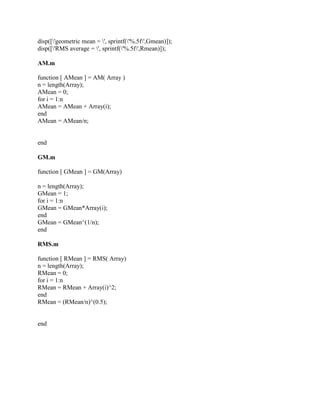Diese Präsentation wurde erfolgreich gemeldet.

# 2- Write a MATLAB program that calculates the arithmetic mean- the geo.docx

Anzeige
Anzeige
Anzeige
Anzeige
Anzeige
Anzeige
Anzeige
Anzeige
Anzeige
Anzeige
Anzeige×

1 von 3 Anzeige

# 2- Write a MATLAB program that calculates the arithmetic mean- the geo.docx

2. Write a MATLAB program that calculates the arithmetic mean, the geometric mean, and the root-mean-square average for a given set of values. Your program must use 3 functions to calculate the 3 required means. The output should be formatted as follows:
Your name Statistical Package arithmetic mean = x.xxxxx geometric mean = x.xxxxx RMS average = x.xxxxx
Test your program on the following values: 1.1, 3.3, 3.00, 2.22, 2.00, 2.72, 4.00, 4.62 and 5.37. Your main program calls 3 functions.
The data should be read by the main program from a text file, stored in an array and then passed to the functions.
The results and any other output should be printed by the main program. Notice how the output results are aligned. Also notice that the results are printed accurate to 5 decimal places.
DO NOT USE MATLAB BUILT-IN FUNCTIONS.
I am stumped on this problem as I am not to good with creating functions in matlab
Solution
I am going to give you 4 files, three functions and one script. Create 4 new scripts in matlab and save them as given. Then run the \"Mean.m\" script.
Mean.m
fid = fopen(\'input.txt\');
Array = fscanf(fid, \'%g\');
Amean = AM(Array);
Gmean = GM(Array);
Rmean = RMS(Array);
display(\'Amlan Das Statistical Package\')
display([\'arithmetic mean = \', sprintf(\'%.5f\',Amean)]);
disp([\'geometric mean = \', sprintf(\'%.5f\',Gmean)]);
disp([\'RMS average = \', sprintf(\'%.5f\',Rmean)]);
AM.m
function [ AMean ] = AM( Array )
n = length(Array);
AMean = 0;
for i = 1:n
AMean = AMean + Array(i);
end
AMean = AMean/n;
end
GM.m
function [ GMean ] = GM(Array)
n = length(Array);
GMean = 1;
for i = 1:n
GMean = GMean*Array(i);
end
GMean = GMean^(1/n);
end
RMS.m
function [ RMean ] = RMS( Array)
n = length(Array);
RMean = 0;
for i = 1:n
RMean = RMean + Array(i)^2;
end
RMean = (RMean/n)^(0.5);
end
.

2. Write a MATLAB program that calculates the arithmetic mean, the geometric mean, and the root-mean-square average for a given set of values. Your program must use 3 functions to calculate the 3 required means. The output should be formatted as follows:
Your name Statistical Package arithmetic mean = x.xxxxx geometric mean = x.xxxxx RMS average = x.xxxxx
Test your program on the following values: 1.1, 3.3, 3.00, 2.22, 2.00, 2.72, 4.00, 4.62 and 5.37. Your main program calls 3 functions.
The data should be read by the main program from a text file, stored in an array and then passed to the functions.
The results and any other output should be printed by the main program. Notice how the output results are aligned. Also notice that the results are printed accurate to 5 decimal places.
DO NOT USE MATLAB BUILT-IN FUNCTIONS.
I am stumped on this problem as I am not to good with creating functions in matlab
Solution
I am going to give you 4 files, three functions and one script. Create 4 new scripts in matlab and save them as given. Then run the \"Mean.m\" script.
Mean.m
fid = fopen(\'input.txt\');
Array = fscanf(fid, \'%g\');
Amean = AM(Array);
Gmean = GM(Array);
Rmean = RMS(Array);
display(\'Amlan Das Statistical Package\')
display([\'arithmetic mean = \', sprintf(\'%.5f\',Amean)]);
disp([\'geometric mean = \', sprintf(\'%.5f\',Gmean)]);
disp([\'RMS average = \', sprintf(\'%.5f\',Rmean)]);
AM.m
function [ AMean ] = AM( Array )
n = length(Array);
AMean = 0;
for i = 1:n
AMean = AMean + Array(i);
end
AMean = AMean/n;
end
GM.m
function [ GMean ] = GM(Array)
n = length(Array);
GMean = 1;
for i = 1:n
GMean = GMean*Array(i);
end
GMean = GMean^(1/n);
end
RMS.m
function [ RMean ] = RMS( Array)
n = length(Array);
RMean = 0;
for i = 1:n
RMean = RMean + Array(i)^2;
end
RMean = (RMean/n)^(0.5);
end
.

Anzeige
Anzeige

### 2- Write a MATLAB program that calculates the arithmetic mean- the geo.docx

1. 1. 2. Write a MATLAB program that calculates the arithmetic mean, the geometric mean, and the root-mean-square average for a given set of values. Your program must use 3 functions to calculate the 3 required means. The output should be formatted as follows: Your name Statistical Package arithmetic mean = x.xxxxx geometric mean = x.xxxxx RMS average = x.xxxxx Test your program on the following values: 1.1, 3.3, 3.00, 2.22, 2.00, 2.72, 4.00, 4.62 and 5.37. Your main program calls 3 functions. The data should be read by the main program from a text file, stored in an array and then passed to the functions. The results and any other output should be printed by the main program. Notice how the output results are aligned. Also notice that the results are printed accurate to 5 decimal places. DO NOT USE MATLAB BUILT-IN FUNCTIONS. I am stumped on this problem as I am not to good with creating functions in matlab Solution I am going to give you 4 files, three functions and one script. Create 4 new scripts in matlab and save them as given. Then run the "Mean.m" script. Mean.m fid = fopen('input.txt'); Array = fscanf(fid, '%g'); Amean = AM(Array); Gmean = GM(Array); Rmean = RMS(Array); display('Amlan Das Statistical Package') display(['arithmetic mean = ', sprintf('%.5f',Amean)]);
2. 2. disp(['geometric mean = ', sprintf('%.5f',Gmean)]); disp(['RMS average = ', sprintf('%.5f',Rmean)]); AM.m function [ AMean ] = AM( Array ) n = length(Array); AMean = 0; for i = 1:n AMean = AMean + Array(i); end AMean = AMean/n; end GM.m function [ GMean ] = GM(Array) n = length(Array); GMean = 1; for i = 1:n GMean = GMean*Array(i); end GMean = GMean^(1/n); end RMS.m function [ RMean ] = RMS( Array) n = length(Array); RMean = 0; for i = 1:n RMean = RMean + Array(i)^2; end RMean = (RMean/n)^(0.5); end